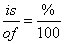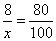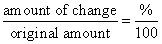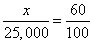##### SATThomas Edison, who invented the light bulb and thus proved that he had some serious brain power, once said, “We don’t know a millionth of one percent about anything.” Edison may have been right when it comes to knowledge in general, but when you take the PSAT/NMSQT, you can easily find the right answer to 100% of the questions involving percents.

A percent is nothing more than a useful way to represent how much of 100 you’re talking about. Because 100% of anything is the whole thing, 50% is half, 25% is a quarter, and so forth. You see percents expressed with the word of, as in 66% of. The of is a stand-in for multiplication. Here’s how to approach percent problems:

• Use your calculator if it has a % button. Some do; some don’t. If you have a % button on your calculator and you need to find 13% of 189, you can simply enter “13% x 189” to see that the answer is 24.57.

No percent button? Just convert the percent to a decimal by moving the decimal point two spaces to the left. In that case you’d enter “0.13 x 189” for the same result. You can also change a percent to a fraction (13% = 13/100).

• Consider plugging in. This PSAT/NMSQT trick, plugging in, is tailor-made for percent problems. Plug in 100 and you’re all set.

• Remember the “is-of” formula. In this formula, you haveso if you’re asked, “80% of what number is 8,” you can see thatNow cross multiply: 80x = 800. Divide by 80 and you get x = 10.

• Pay special attention to problems asking for the percent of increase or decrease. Plugging in helps here also, or you can use this formula:• Don’t forget that percentages may be more than 100. You’d probably like your allowance to increase by 300% (so you’d pocket 4 times as much money), but it’s more likely that your college tuition will rise 500% by the time you graduate.

1. Jane goes to get her hair cut and tells the person cutting her hair that she would like her hair to be 25% shorter than it currently is. After the haircut, Jane’s hair is 12 inches long. How many inches long was Jane’s hair before the haircut?

(A) 9
(B) 12
(C) 14
(D) 16
(E) 18
2. A bookstore sells books for 20% more than it buys them for. During a sale, a customer buys a book with a 25% discount coupon. What percent of the price that the store paid did the book sell for?

(A) 60%
(B) 75%
(C) 90%
(D) 100%
(E) 110%
3. Forty percent of the seats at a baseball stadium are in the shade at 4 p.m. If the stadium has 25,000 seats, how many seats are in the sun at 4 p.m.?

(A) 5,000
(B) 10,000
(C) 12,500
(D) 15,000
(E) 20,000

1. D. 16

Plugging in is a great strategy for solving this problem. If Jane began with 14 inches of hair — Choice (C) — then after the haircut she’ll still have 75% of that length: 14(0.75) = 10.5 inches. Jane has more hair left than that, so pick a longer starting length.

If Jane began with 16 inches of hair, Choice (D), then she’d have 16(0.75) = 12 inches of hair after the cut — the answer you’re looking for!

2. C. 90%

As usual, you want to use 100 as your initial amount in a percent problem, even if it sounds silly for a book to cost \$100. That means that the customers saw the price as 20% or \$20 higher: \$120. A 25% discount is the same as paying 75% of the price, so the sale price is 120(0.75) = \$90.

\$90 is 90% of \$100, so Choice (C) is the one you want.

3. D. 15,000

If 40% of the seats are in the shade, then 60% of them are in the sun. To find out how many seats are in the sun, you want to know what number is 60% of 25,000. Using the formulaand letting x be the number you’re looking for:Cross-multiply and simplify, and you see that x = 15,000, Choice (D).